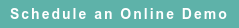# RoyaltyStat Blog

### Posts by Topic

Y si pretendes remover las ruinas que tú mismo hiciste ...

Cenizas sung by Toña La Negra. Bolero lyrics by Wello Rivas (1913-1990).

We suggested on prior blogs that operating assets (measured by property, plant & equipment) are endogenous and that structural equation estimates of return on assets produce biased coefficients. Here, we provide another alternative from biased estimates of return on assets than using exotic algorithms like two-stage least squares.

We suggested also that structural equations must belong to an accounting system, and that we need to consider the appropriate accounting identity and estimate reduced form equations. In what follows, we examine the return on assets equation if or when assets are exogenous.

We start with a given company (enterprise) account identity equation, which holds true by definition:

##### (1)     S(t) = C(t) + δ K(t – 1) + P(t)

in which the variable S(t) denotes net sales (revenue) in year t = 1 to T, C(t) denotes total cost (XOPR), combining cost of goods (COGS) and operating expenses (XSGA), the parameter δ denotes the annual depreciation rate of K(t – 1), property, plant & equipment (PPENT), taken stock at the beginning of the year, and P(t) denotes operating profits after depreciation, excluding the amortization of intangibles (OAIDP + AM).

We introduce a behavioral equation that operating profits are proportional to operating assets at the beginning of the year, plus a random error:

##### (2)     P(t) = ρ K(t – 1) + U(t)

Other behavioral equations different from (2) are plausible, and rival economic schools are formed over nonconcur behavioral equations.

We restrict operating assets to property, plant & equipment because this is the only composite balance sheet account subject to annual depreciation allowance.

Operating assets such as cash & equivalents, accounts receivable, and inventory are not subject to annual depreciation from wear and tear; therefore, they should not be combined with depreciable assets.

The dependent variable of equation (2) includes δ K(t – 1), which is depreciation in the beginning of the period, and we must move this variable to the right-hand side when we combine equations (1) and (2).

We combine equations (1) and (2) and obtain a single reduced form equation, which we can subject to statistical (regression) analysis:

##### (3)     Z(t) = φ K(t – 1) + U(t)

where Z(t) =[S(t) − C(t)] denotes operating profits before depreciation and amortization (OIBDP) and φ = (δ + ρ) > 0 includes the desired return on assets.

After we consider the accounting identity (1), the dependent variable of the regression equation (3) is changed from P(t) to Z(t). We need to know the depreciation rate to find the return on assets, because the regression slope coefficient combines both the annual depreciation rate and the annual return on assets.

We examined 17 years of data for several major U.S. retailers from 2002 (after the Dotcom debacle) to 2019, and obtained disappointing results from equation (3). The regression results suggest that assets are not significant profit drivers for major retailes:

 Company GVKEY φ t-statistics R2 Best Buy 2184 0.4882 3.4201 0.4076 Conn’s Inc. 156614 0.789 3.4788 0.4272 Costco 29028 0.3685 47.669 0.9899 Home Depo* 5680 −0.5299 −0.8658 0.1279 Kohl’s 25283 0.1856 6.8047 0.6972 Lowe’s* 6829 0.0698 0.6864 0.0471 Macy’s* 4611 0.1088 1.6856 0.1181 Target 3813 0.1215 3.0819 0.494 Walmart 11259 0.2337 11.0817 0.8838

The regression coefficients of Home Depot, Lowe’s, and Macy’s (companies with an asterisk superscript attached to their names) are not significant, and the positive or negative values can result from random fluctuations. The t-statistics are produced using Newey-West correction. The low R2 reveal the poor fit of equation (3) for most retailers' data. The regession slope coefficient of Home Depot is perverse, not showing the expected positive sign.

The discordant regression results of the table above show that return on assets is not an appropriate profit indicator to measure the arm’s length nature of related party transactions among major U.S. retailers. Here, we get more confirmation that the profit markup equation that we expounded on other blogs is unbeatable to provide benchmarks for related party transactions in services, distribution and retail. See https://blog.royaltystat.com/transfer-pricing-methods-based-on-operating-profits

Published on Apr 28, 2020 1:54:36 PM

Ednaldo Silva (Ph.D.) is founder and managing director of RoyaltyStat. He helped draft the US transfer pricing regulations and developed the comparable profits method called TNNM by the OECD. He can be contacted at: esilva@royaltystat.com

RoyaltyStat provides premier online databases of royalty rates extracted from unredacted license agreements
and normalized company financials (income statement, balance sheet, cash flow). We provide high-quality data, built-in analytical tools, customer training and attentive technical support.